## Solutions of Magnetic Effects of Electric Current Lakhmir Singh Manjit Kaur  SAQ, LAQ, HOTS and MCQ Pg No. 82 Class 10 Physics12. Describe how you will locate a current-carrying wire concealed in a wall.

→ To locate a current carrying wire concealed in a wall one should use a plotting compass. by moving plotting compass on the wall the deflection in its needle shows the place where wire is concealed in the wall.

13. Describe some experiment to show that the magnetic field is associated with an electric current.Let us take a copper wire AB on a plane such that it faces North-South. Now place a plotting compass under it. connect both end to a battery with a switch to put it on and off.
When the switch is on then the plotting compass needle shows deflection. When its turned off then the compass stop showing any deflection. This shows that the magnetic field is associated with electric field.

14A. Draw a sketch to show the magnetic lines of force due to a current-carrying straight conductor.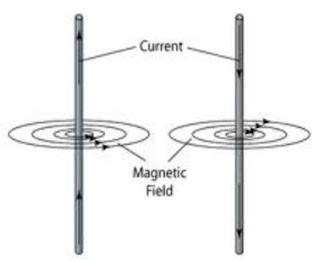14B. Name and state the rule to determine the direction of magnetic field around a straight current-carrying conductor.

→ The Fleming's left rule is used to determine the direction of magnetic field around a straight current-carrying conductor. According to the rule,
The F(Thumb) represents the direction of Force of the conductorThe B(Forefinger) represents the direction of the Magnetic fieldThe I(Centre finger) represents the direction of the Current.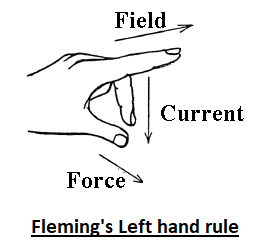15. State and explain Maxwell's right-hand thumb rule.

→ Maxwell’s right hand thumb rule. According to this law if we hold our thumb of right hand in the direction of magnetic field and close our finger then our enclosed finger shows the magnetic field line around the conductor.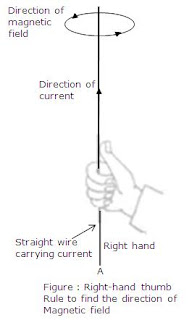Let AB be a straight wire. Placed vertically A to B. To find the direction of magnetic field we assume that we are holding the current carrying wire in such a way that our thumb of right hand shows the direction of flow of current. Then the finger enclosing the wire. The direction in which our finger is enclosed shows the direction of magnetic field which is anticlockwise.

16. What is Maxwell's corkscrew rule? For what purpose is it used?

→ Maxwell’s right hand thumb rule is also known as Maxwell’s corkscrew rule. It is used to find out the direction of magnetic field along a straight current carrying conductor.

17A. Draw the magnetic lines of force due to a circular wire carrying current.17B. What are the various ways in which the strength of magnetic field produced by a current-carrying circular coil can be increased?

→ There are two ways of doing this :-
(1) must increase the number of turns of the coil.
(2) Must increase the current flow in the coil.

18. State and explain the Clock face rule for determining the polarities of a circular wire carrying current.

→ According to this rule, we first look at one face of the coil and if the current through the coil seems to be flowing in the clockwise direction, then that face is considered as the South pole. And if the current seems to be flowing in the anti-clockwise direction, then the face of the coil is considered as the North pole.
a) For this face of the coil, the current is flowing in the clockwise direction, so it acts as South-Pole.
b) For this face of the coil, he current if flowing in he anti-clockwise direction, so it acts as North-Pole.19. Name any two factors on which the strength of magnetic field produced by a current-carrying solenoid depends. How does it depend on these factors?

→ It depends on following factors:-
i) Current: Larger he current, stronger will be the magnetic field.
ii) Number of coils in the solenoid: Greater the number of coils in the solenoid, stronger will be the magnetic field produced.

20A. Draw a circuit diagram to show how a soft iron piece can be transformed into an electromagnet.

→ A coil C of insulated wire is wound around the soft iron core and the two ends of the coil are connected to a battery. This is how an electromagnet is made.20B. Describe how an electromagnet could be used to separate copper from iron in a scrap yard.

→ Electromagnetic cranes are used to separate copper from iron in a scrap yard. Switch in on the curen energises the electromagnet, thus producing magnetic field. This magnetic field attracts the iron pieces, thus separating them from the copper.

21A. How does an electromagnet differ from a permanent magnet?

→ An electromagnet produces a temporary magnetic field which sustains till the current flows through the coils of the electromagnet, while a permanent magnet produces a permanent magnetic field.

21B. Name two devices in which electromagnets are used and two devices where permanent magnets are used.

→ Electromagnets are used in Electric bell and Electric motors.
Permanent magnets are used in Refrigerators doors and Toys.

22A. What is a solenoid? Draw a sketch to show the magnetic field pattern produced by a current-carrying solenoid.

→ A solenoid is a long coil containing a large number of close turns of insulated copper wire.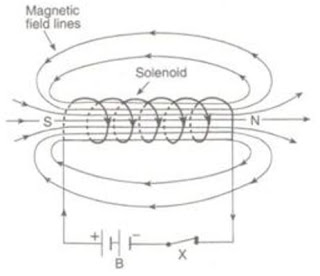22B. Name the type of magnet with which the magnetic field pattern of a current-carrying solenoid resembles.

→ The magnetic field produced by a current carrying solenoid is similar to that produced by a bar magnet.

22C. What is the shape of field lines inside a current-carrying solenoid? What does the pattern of field lines inside a current-carrying solenoid indicate?

→ Magnetic field lines inside a current carrying conductors are in the form of parallel straight lines.

22D. List three ways in which the magnetic field strength of a current-carrying solenoid can be increased?

→ Following are the three ways of increasing the strength of the magnetic field of current carrying solenoid:
i) Increasing the current flowing through the coil of solenoid.
ii) Increasing the number of turns in he solenoid.

22E. What type of core should be put inside a current-carrying solenoid to make an electromagnet ?

→ Soft iron core should be used.

23A. What is an electromagnet? Describe the construction and working of an electromagnet with the help of a labelled diagram.

→ An electromagnet is called a temporary magnet because it produces magnetic field lines only when the current flows through the coil of the electromagnet. When we stop the current through the coil, the magnetic field lines diminishes.

23B. Explain why, an electromagnet is called a temporary magnet.

→ Soft iron core is used in the electromagnets because it loses all its magnetism when the current through the coil stops flowing. While in case of steel, there is still some magnetism even after the current stops flowing through the coil of electromagnet.

23C. Explain why, the core of an electromagnet should be of soft iron and not of steel.

→ The strength of electromagnet depends on the following factors:
i) Number of turns in the coil: Increasing the number of turns in the coil increase the strength of magnetic field produced by the electromagnet.
ii) Current flowing through the coil: Increasing the current flowing through the coil increases the strength of magnetic field produced.
iii) Length of air gap between its poles: Decreasing he length of air gap between the poles of electromagnet increases the strength of electromagnet.

23D. State the factors on which the strength of an electromagnet depends. How does it depend on these factors ?

→ An electromagnet is a temporary magnet which works on the magnetic effect of the current. It consists of long coil of insulated copper wire wound around a soft iron core and he two ends of he coil are connected to a battery. As the current flows through the coil, the magnetic field lines ae produced. These field lines sustains till the current continues to flow through the coil of electromagnet.

23E. Write some of the important uses of electromagnets

→ Electromagnets are used in several devices such as Electric bell, Electric motors, Loudspeakers, etc. They are also used by doctors to remove iron or steel particles from patient’s eyes and to remove iron pieces from wounds.

Multiple Choice Questions (MCQs)-Pg-83

24. The strength of the magnetic field between the poles of an electromagnet would be unchanged if :
A. current in the electromagnet winding were doubled
B. direction of current in electromagnet winding were reversed
C. Distance between the poles of electromagnet were doubled
D. material of the core of electromagnet were changed

→ The strength of the magnetic field between the poles of an electromagnet would be unchanged if the direction of current in electromagnet winding were reversed.

25. The diagram given below represents magnetic field caused by a current-carrying conductor which is:A. a long straight wire
B. a circular coil
C. a solenoid
D. a short straight wire

→ The diagram represents the magnetic field caused by a circular current carrying conductor.

26. The magnetic inside a long straight solenoid carrying current :
A. is zero
B. decreases as we move towards its end.
C. increases as we move towards its end.
D. is the same at all points.

→ The magnetism inside a long straight solenoid remains same at every point.

27. Which of the following correctly describes the magnetic field near a long straight wire?
A. The field consists of straight lines perpendicular to the wire.
B. The field consists of straight lines parallel to the wire.
C. The field consists of radial lines originating from the wire.
D. The field consists of concentric circles centred on the wire.

→ The magnetic field near a long straight wire consists of concentric circles centred on the wire.

28. The north-south polarities of an electromagnet can be found easily by using:
A. Fleming’s right-hand rule
B. Fleming’s left-hand rule
C. Clock face rule
D. Left-hand thumb rule

→ The north-south polarities of an electromagnet can be found easily by using clock face rule.

29. The direction of current in the coil at one end of an electromagnet is clockwise. This end of the electromagnet will be:
A. north pole
B. east pole
C. south pole
D. west pole

→ The direction of current in the coil at one end of an electromagnet is clockwise then that end is south pole.

30. If the direction of electric current in a solenoid when viewed from a particular end is anticlockwise, then this end of solenoid will be:
A. west pole
B. south pole
C. north pole
D. east pole

→ The direction of electric current is anticlockwise when viewed form the north pole.

31. The most suitable material for making the core of an electromagnet is:
A. soft iron
B. brass
C. aluminium
D. steel

→ Soft iron is mostly used for making the core of the electromagnet.

32. The magnetic effect of current was discovered by:
A. Maxwell
B. Fleming
C. Oersted

→ The magnetic effect of the current was discovered by Oersted.

33. A soft iron bar is inserted inside a current-carrying solenoid. The magnetic field inside the solenoid:
A. will decrease
B. will increase
C. will become zero
D. will remain the same

→ When soft iron bar is inserted inside a current carrying solenoid then it increases the magnetic field inside the solenoid.

34. The magnetic field lines in the middle of the current-carrying solenoid are :
A. circles
B. spirals
C. parallel to the axis of the tube
D. perpendicular to the axis of the tube

→ The magnetic field lines in the middle of the current carrying solenoid are parallel to the axis of the tube.

35. The front face of a circular wire carrying current behaves like a north pole. The direction of current in this face of the circular wire is:
A. clockwise
B. downwards
C. anticlockwise
D. upwards

→ When the front face of a circular wire carrying current behaves like north pole ,then the direction of current in this field is anticlockwise.

36. The back face of a circular loop of wire found to be south magnetic pole. The direction of current in this face of the circular loop of wire will be:
A. towards south
B. clockwise
C. anticlockwise
D. towards north

→ The back face of a circular loop of wire found to be south magnetic pole .the direction of the current in this face is clockwise.

Questions Based on High Order Thinking Skills (HOTS)-Pg-84

37. In the straight wire A, current is flowing in the vertically downward direction whereas in wire B the current is flowing in the vertically upward direction. What is the direction of magnetic field:
(a) in wire A?
(b) in wire B?
Name the rule which you have used to get the answer.

→ (a) Clockwise would be the direction of magnetic field in wire A.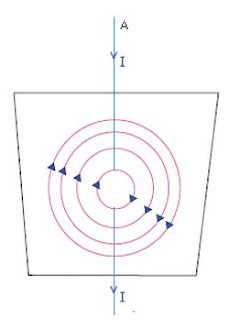(b) the direction of the magnetic field in the wire B is anticlockwise.Maxwell’s right hand thumb rule.

38. The figure shows a solenoid wound on a core of soft iron. Will the end A be a N pole or S pole when the current flows in the direction shown?→ According to the fig. end a is the south pole as the direction of the current flow is clockwise from this end.

39. A current-carrying straight wire is held in exactly vertical position. H the current passes through this wire in the vertically upward direction, what is the direction of magnetic field produced by it? Name the rule used to find out the direction of magnetic field.

→ The direction of the current flow is anticlockwise. This can be determined by using Maxwell’s right hand thumb rule.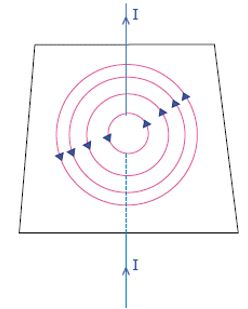40. For the coil in the diagram below, when the switch is pressed:
(a) What is the polarity of end A?
(b) Which way will the compass point then?→ (a) End A becomes south pole. This is because from the end at the current flows in clockwise direction.
(b) The movement of the compass needle is away form the end B. As end B is the north pole.

41. A current flows downwards in a wire that passes vertically through a table top. Will the magnetic field lines around it go clockwise or anticlockwise when viewed from above the table?

→ According to the Maxwell’s right hand thumb rule the direction of the magnetic field will be in clockwise direction.

42. The directions of current flowing in the coil of an electromagnet at its two ends X and Y are as shown below:(a) What is the polarity of end X?
(b) What is the polarity of end Y?
(c) Name and state the rule which you have used to determine the polarities.

→ The easy way to find the polarity is right-hand rule: " If the current flows in the direction of your fingers, the thumb points towards the north pole".If we assume an electromagnet which has two ends i.e. X and Y, then,
(a) South is the polarity of end X as direction of current flow from that end is clockwise.
(b) The end Y will be the north pole as the current flow from this end shows the anticlockwise direction.
(c) The rule used here to find the polarity of the ends is clock face rule. According to this rule if the face from where it is being observed is showing the flow of current in anticlockwise direction then that end is N-pole but if the same face shows the flow of current in a clockwise direction then that end becomes S-pole.

43. The magnetic field associated with a current-carrying straight conductor is in anticlockwise direction. H the conductor was held along the east-west direction, what will be the direction of current through it? Name and state the rule applied to determine the direction of current?

→ East to west will be the direction of the current.
According to the Maxwell’s right hand thumb rule if the enclosed fingers shows the direction of the magnetic field then the thumb will always point in the direction of current flow.

44. A current-carrying conductor is held in exactly vertical direction. In order to produce a clockwise magnetic field around the conductor, the current should be passed in the conductor:
(a) from top towards bottom
(b) from left towards right
(c) from bottom towards top
(d) from right towards left

→ (a) the current should be passed from top to bottom to produce a clockwise magnetic field around the conductor.

45. A thick wire is hanging from a wooden table. An anticlockwise magnetic field is to be produced around the wire by passing current through this wire by using a battery. Which terminal of the battery should be connected to the:
(a) top end of wire?
(b) bottom end of wire?

→ The direction desired to pass the current is upward.

1. What happens when a current-carrying conductor is placed in a magnetic field?

→ When a conductor is placed in a magnetic field then a mechanical force is exerted on the conductor, which eventually make the conductor move.

2. When is the force experienced by a current-carrying conductor placed in a magnetic field largest ?

→ The force experienced by a current carrying conductor is largest when it is placed at right angle in a magnetic field.

3. In a statement of Fleming's left-hand rule, what do the following represent?
(a) direction of centre finger.
(b) direction of forefinger.
(c) direction of thumb.

→ (a) By Fleming’s left hand rule direction of centre finger represent the current.
(b) Direction of the forefinger in the Fleming’s left hand rule represents the magnetic field.
(c) Force acting on the conductor is represented by the direction of thumb in Fleming’s left hand rule.

4. Name one device which works on the magnetic effect of current.

→ The device which work on the magnetic effect of current is electric bell. Electric bell works when electromagnetism is produced.

5. Name the device which converts electrical energy into mechanical energy.

→ Electrical motor is used to convert electrical energy in to mechanical energy.

6. A motor converts one form of energy into another. Name the two forms.

→ A motor converts electrical energy into mechanical energy. So the two forms are electrical and mechanical.

7. State whether the following statement is true or false:
An electric motor converts mechanical energy into electrical energy.

→ False, because electric motor is used to convert electrical energy in to mechanical energy.

8. For Fleming's left-hand rule, write down the three things that are 90° to each other, and next to each one write down the finger or thumb that represents it.

→ The three things that are 90° to each other, and next to each, and next to each one are:-
Current- it is denoted by the direction of the centre finger.
Magnetic field- ii is denoted by the direction of the fore finger.
Force or motion- it is denoted by the thumb.

9. Name the device which is used to reverse the direction of current in the coil of a motor.

→ Commutator is used to reverse the direction of current in the coil of a motor.

10. What is the other name of the split ring used in an electric motor?

→ Commutator is the other name for the split ring which is used in the coil of a motor.

11. What is the function of a commutator in an electric motor?

→ Its function is to reverse the current every time it reaches the vertical position in the coil.

12. Of what substance are the brushes of an electric motor made ?

The brushes of an electrical motor are made of carbon.

13. Of what substance is the core of the coil of an electric motor made?

→ The core of the coil of an electric motor is made of soft iron.

14. In an electric motor, which of the following remains fixed and which rotates with the coil?

→ The part of the electric motor which remain fixed is brush and the part which rotate with the coil is commutator.

15. What is the role of the split ring in an electric motor?